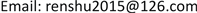1. 引言

2. 超几何分布模型建立

2.1. 随机变量元素d = 1, 2, ∙∙∙, n的超几何模型推导

L d 1 = C D d 1 C N − D n − d 1 C N n = ( N − D ) ! N ! ⋅ ( N − n ) ! ( N − D − n + d 1 ) ! ⋅ n ! ( n − d 1 ) ! ⋅ D ! d 1 ! ( D − d 1 ) !         d 1 = 1 , 2 , ⋯ , n (1)

L d 1 = ( N − D ) ! ( N − D ) !   ⋅ ( N − D + 1 ) ⋅ ( N − D + 2 ) ⋅ ⋅ ⋅ N     ⋅ [ ( N − D ) − ( n − d 1 ) ] !   ⋅ ( N − D − n + d 1 + 1 ) ⋅ ( N − D − n + d 1 + 2 ) ⋅ ⋅ ⋅ ( N − n ) [ ( N − D ) − ( n − d 1 ) ] !     ⋅ ( n − d 1 ) !   ⋅ ( n − d 1 + 1 ) ⋅ ( n − d 1 + 2 ) ⋅ ⋅ ⋅ n ( n − d 1 ) ! ⋅ ( n − d 1 ) ! ⋅ ( n − d 1 + 1 ) ⋅ ( n − d 1 + 2 ) ⋅ ⋅ ⋅ n ( n − d 1 ) !   ⋅ d 1 ! = ( 1 − n − d 1 N − D + 1 ) ⋅ ( 1 − n − d 1 N − D + 2 ) ⋅ ⋅ ⋅ ( 1 − n − d 1 N − D + D − d 1 ) ⋅ ( 1 − N − n N − d 1 + 1 )     ⋅ ( 1 − N − n N − d 1 + 2 ) ⋅ ⋅ ⋅ ( 1 − N − n N − d 1 + d 1 ) ⋅ ( 1 + D − d 1 1 ) ⋅ ( 1 + D − d 1 2 ) ⋅ ⋅ ⋅ ( 1 + D − d 1 d 1 )

L d 1 = ∏ k = 1 D − d ( 1 − n − d N − D + k ) ⋅ ∏ k = 1 d ( 1 − N − n N − d + k ) ⋅ ∏ k = 1 d ( 1 + D − d k )         d = 1 , 2 , 3 , ⋯ , n (2)

2.2. 特殊随机变量d = 0的超几何分布模型

L d 2 = C N − D n C N n = ( N − D ) N ! ⋅ ( N − n ) ! ( N − D − n ) ! (3)

L d 2 = ∏ k = 1 D ( 1 − n N − D + k ) (4)

2.3. 超几何分布完整模型

L d = C D d C N − D n − d C D n = { ∏ k = 1 D − d ( 1 − n − d N − D + k ) ⋅ ∏ k = 1 d ( 1 − N − n N − d + k ) ⋅ ∏ k = 1 d ( 1 + D − d k )           d = 1 , 2 , ⋯ , n ∏ k = 1 D ( 1 − n N − D + k )                                                                                                                 d = 0 (5)

3. 结束语